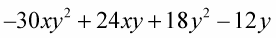##### Basic Math & Pre-Algebra For DummiesDistributing variables over the terms in an algebraic expression involves multiplication rules and the rules for exponents. When different variables are multiplied together, you can write them side by side without using any multiplication symbols. If the same variable is multiplied as part of the distribution, then you add the exponents.

The exponent rule says that when multiplying exponents with the same base, you add the exponents: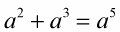This sample problem illustrates the exponent rule for multiplying terms with the same base.

1. Distribute the term outside the parentheses over the terms within.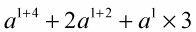3. Complete the distribution.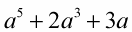The next example contains negative powers and fractional powers — the rules for exponents remain the same with negative and fractional exponents.

Example: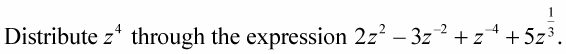1. Distribute the term outside the parentheses over the terms within the parentheses.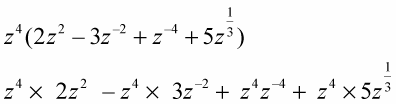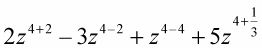3. Complete the distribution.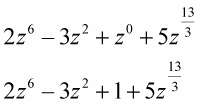The exponent zero means the value of the expression is one: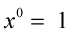You combine exponents with different signs by using the rules for adding and subtracting signed numbers. Fractional exponents are combined after finding common denominators. Exponents that are improper fractions are left in that form.

Try going through many of the situations that could arise when distributing, such as distributing both a number and a variable, distributing various powers of more than one variable, distributing negatives, rewriting negative exponents as fractional terms, distributing fractional powers, and distributing radicals. This touches on just about anything you’d probably come across.

Combine the variables by using the rules for exponents.

Example 1: Distribute 5x through the expression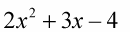Multiply each term by 5x.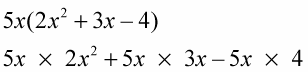Then multiply the numbers and the variables in each term.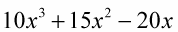Example 2: Combine the variables with the same base using the rules for exponents. The signs of the results follow the rules for multiplying signed numbers.

–6y(5xy – 4x – 3y + 2)

Multiply each term by -6y.

–6y(5xy) – 6y(–4x) – 6y(–3y) – 6y(2)

Do the multiplication in each term.